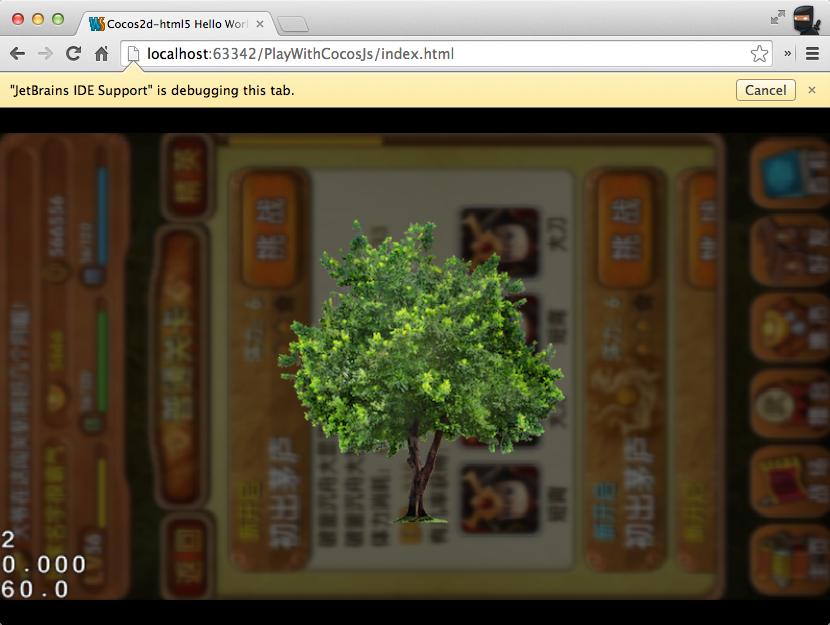# 木匣子

Web/Game/Programming/Life etc.

# Cocos2d-js 实现弹窗背景虚化效果

### ¶RenderTexture/FrameBuffer

``````var bgTex = cc.RenderTexture.create(cc.visibleRect.width, cc.visibleRect.height);
bgTex.begin();
cc.director.getRunningScene().visit();
bgTex.end();
``````

### ¶Software Blur

• StackBox Blur: Box Blur 的升级版，效果更好一些，而且速度是 Gaussian Blur 的 7 倍；

Box Blur 的效果过于生硬，而 Gaussian Blur 的效果好很多。

``````//apply blurring, using a 15-tap filter with predefined gaussian weights
sum += texture2D(CC_Texture0, vec2(tc.x - 7.0*blur*hstep, tc.y - 7.0*blur*vstep)) * 0.0044299121055113265;
sum += texture2D(CC_Texture0, vec2(tc.x - 6.0*blur*hstep, tc.y - 6.0*blur*vstep)) * 0.00895781211794;
sum += texture2D(CC_Texture0, vec2(tc.x - 5.0*blur*hstep, tc.y - 5.0*blur*vstep)) * 0.0215963866053;
sum += texture2D(CC_Texture0, vec2(tc.x - 4.0*blur*hstep, tc.y - 4.0*blur*vstep)) * 0.0443683338718;
sum += texture2D(CC_Texture0, vec2(tc.x - 3.0*blur*hstep, tc.y - 3.0*blur*vstep)) * 0.0776744219933;
sum += texture2D(CC_Texture0, vec2(tc.x - 2.0*blur*hstep, tc.y - 2.0*blur*vstep)) * 0.115876621105;
sum += texture2D(CC_Texture0, vec2(tc.x - 1.0*blur*hstep, tc.y - 1.0*blur*vstep)) * 0.147308056121;
sum += texture2D(CC_Texture0, vec2(tc.x, tc.y)) * 0.159576912161;
sum += texture2D(CC_Texture0, vec2(tc.x + 1.0*blur*hstep, tc.y + 1.0*blur*vstep)) * 0.147308056121;
sum += texture2D(CC_Texture0, vec2(tc.x + 2.0*blur*hstep, tc.y + 2.0*blur*vstep)) * 0.115876621105;
sum += texture2D(CC_Texture0, vec2(tc.x + 3.0*blur*hstep, tc.y + 3.0*blur*vstep)) * 0.0776744219933;
sum += texture2D(CC_Texture0, vec2(tc.x + 4.0*blur*hstep, tc.y + 4.0*blur*vstep)) * 0.0443683338718;
sum += texture2D(CC_Texture0, vec2(tc.x + 5.0*blur*hstep, tc.y + 5.0*blur*vstep)) * 0.0215963866053;
sum += texture2D(CC_Texture0, vec2(tc.x + 6.0*blur*hstep, tc.y + 6.0*blur*vstep)) * 0.00895781211794;
sum += texture2D(CC_Texture0, vec2(tc.x + 7.0*blur*hstep, tc.y + 7.0*blur*vstep)) * 0.0044299121055113265;
``````

### ¶LayerBlur

``````var modalBg = LayerBlur.create(LayerBlur.defaultBlurRadius, cc.color(0, 0, 0, 255 * 0.6));
this.addChild(modalBg);
``````UPDATED 20140528

• cocos2d-x 中须使用独立 shader，且需要使用两次 bgTex 缓冲区；
• cocos2d-x 在 visit() 渲染包含 ccui 的组件时，会产生奇怪的 bug 导致场景被色块覆盖。

UPDATED 20141031: